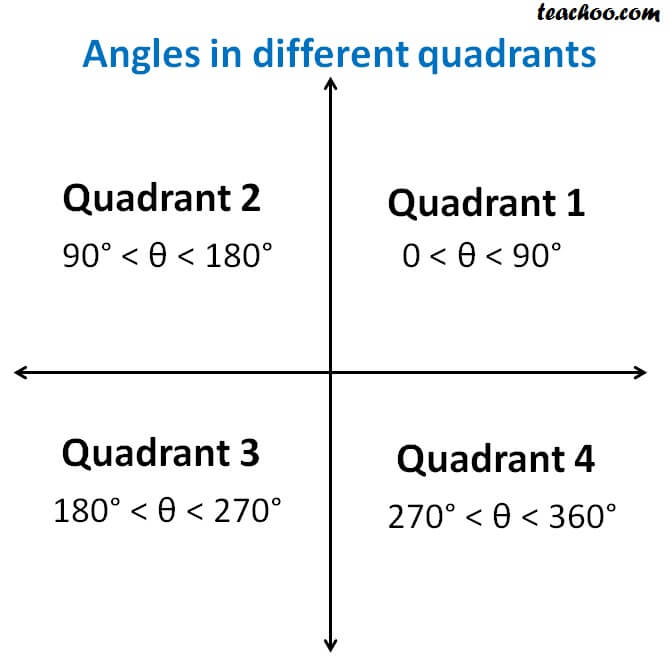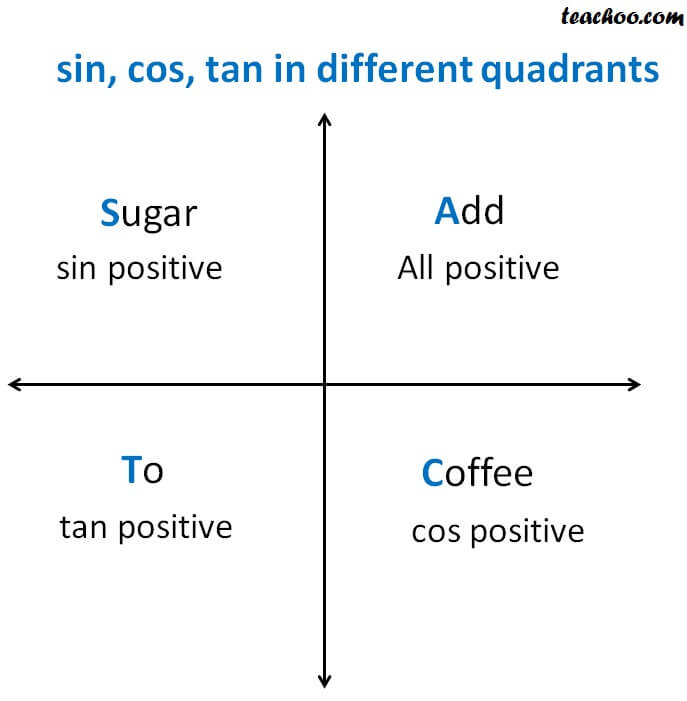Finding Value of trignometric functions, given other functions

Chapter 3 Class 11 Trigonometric Functions
Concept wise

Let’s see the angles in different Quadrants

• In Quadrant 1 , angles are from 0 to 90°
• In Quadrant 2 , angles are from 90 to 180°
• In Quadrant 3 , angles are from 180° to 270°
• In Quadrant 4 , angles are from 270 to 360°To learn sign of sin, cos, tan in different quadrants,

we remember

A dd → S ugar → T o → C offee## Representing as a table

 Quadrant I Quadrant II Quadrant III Quadrant IV sin + + – – cos + – – – tan + – + –

## What about cot, sec, cosec?

We know that

tan = sin/cos

sec = 1/cos

cosec = 1/sin

cot = 1/tan

So,

• In 1 st quadrant -
sin, cos both +ve.

tan = sin/cos = +/+ = +
cosec = 1/sin = 1/+ = +
sec = 1/cos = 1/+ = +
cot = 1/tan = 1/+ = +

• In 2 nd quadrant -
sin is +ve, cos is –ve.

tan = sin/cos = +/– = –
cosec = 1/sin = 1/+ = +
sec = 1/cos = 1/– = –
cot = 1/tan = 1/– = –

• In 3 rd quadrant -
sin is –ve, cos is –ve.

tan = sin/cos = –/– = +
cosec = 1/sin = 1/– = –
sec = 1/cos = 1/– = -
cot = 1/tan = 1/+ = +

• In 4 th quadrant -
sin is –ve, cos is +ve.

tan = sin/cos = –/+ = –
cosec = 1/sin = 1/– = –
sec = 1/cos = 1/+ = +
cot = 1/tan = 1/– = –

Learn in your speed, with individual attention - Teachoo Maths 1-on-1 Class

### Transcript